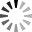# c# API to calculate parabolic sar

c# API to calculate parabolic sarHi,
I'm trying to use the parabolic sar class in the S# library inside my C# program. My understanding is I need to populate the SAR object with values using the process() method like this:

Code

ParabolicSar psar = new ParabolicSar
{
Acceleration = SARSeed,
AccelerationStep = 0.02M,
AccelerationMax = 0.2M
};
for(int i = 0; i < iBars; i++)
{
TimeFrameCandle candle = new TimeFrameCandle()
{
ClosePrice = (decimal)closeArr[i],
HighPrice = (decimal)highArr[i],
LowPrice = (decimal)lowArr[i],
OpenPrice = (decimal)openArr[i],
};
psar.Process(candle);
}

var sarValue = psar.GetValue(shift);

But I am getting no values and the container is empty. How can I fill it with data to get the correct PSAR value at a specific candle instance. Your help is appreciated.

Thank you

 Thanks:

Attach files by dragging & dropping, , or pasting from the clipboard.